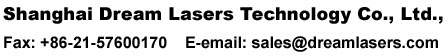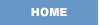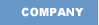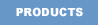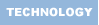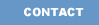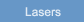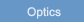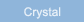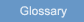Sum Frequency Generation

Differential-Frequency Generation

Optical Parametric Generation

Phase Matching

Conversion Efficiency

Angle Tilting

Optimum Crystal Cut

Crystal Size

Brewster's angle

Handle A NLO Crystal

 Sum Frequency Generation (SFG) w1 + w2 = w3 (or 1/l1 + 1/l2 = 1/l3 in wavelength) It combines two low-energy (or low- frequency) photons into a high-energy photon. For example: 　1064nm + 532nm -----> 355nm Frequency Doubling Frequency Doubling or Second Harmonic Generation (SHG) is a special case of sum frequency generation if the two input wavelengths are the same: 2w1 = w2 (or l1 = 2l2 in wavelength). The simplest scheme for frequency doubling is extracavity doubling. The laser passes through the nonlinear crystal only once as shown. However, if the power density of laser is low, focused beam, intracavity doubling and external resonant cavity are normally used to increase the power density on the crystals, for example, for doubling of cw Nd:YAG laser and Argon Ion lasers.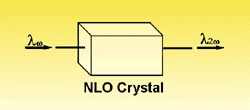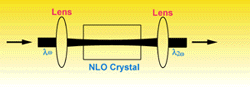Extracavity SHG SHG With Focused Beam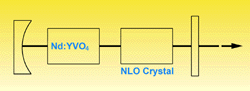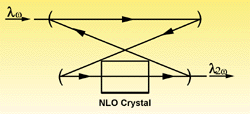Intracavity SHG External Resonant cavity SHG Frequency Tripling Frequency Tripling or Third Harmonic Generation (THG) is an other example of Sum Frequency Generation where, for THG of Nd:YAG laser, l1 = 1064nm, l2 = 532nm and generated wavelength l3 = 355nm. By sum frequency of fundamental wavelength and THG of a Ti:Sapphire laser in BBO crystal, it can generate wavelength as short as 193nm.
 Home | Company Profile | Lasers | Optics | Crystals | Support | Constact Copyright © 2002-2008 by Shanghai Dream Lasers Technology Co., Ltd.